mscroggs.co.uk
mscroggs.co.uksubscribe

# Blog

2018-07-07
So you've calculated how much you should expect the World Cup sticker book to cost and recorded how much it actually cost. You might be wondering what else you can do with your sticker book. If so, look no further: this post contains 5 mathematical things involvolving your sticker book and stickers.

### Test the birthday paradox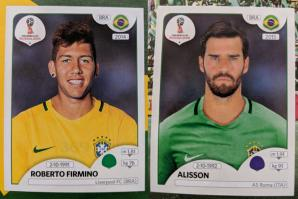Stickers 354 and 369: Alisson and Roberto Firmino
In a group of 23 people, there is a more than 50% chance that two of them will share a birthday. This is often called the birthday paradox, as the number 23 is surprisingly small.
Back in 2014 when Alex Bellos suggested testing the birthday paradox on World Cup squads, as there are 23 players in a World Cup squad. I recently discovered that even further back in 2012, James Grime made a video about the birthday paradox in football games, using the players on both teams plus the referee to make 23 people.
In this year's sticker book, each player's date of birth is given above their name, so you can use your sticker book to test it out yourself.

### Kaliningrad

One of the cities in which games are taking place in this World Cup is Kaliningrad. Before 1945, Kaliningrad was called Königsberg. In Königsburg, there were seven bridges connecting four islands. The arrangement of these bridges is shown below.
The people of Königsburg would try to walk around the city in a route that crossed each bridge exactly one. If you've not tried this puzzle before, try to find such a route now before reading on...
In 1736, mathematician Leonhard Euler proved that it is in fact impossible to find such a route. He realised that for such a route to exist, you need to be able to pair up the bridges on each island so that you can enter the island on one of each pair and leave on the other. The islands in Königsburg all have an odd number of bridges, so there cannot be a route crossing each bridge only once.
In Kaliningrad, however, there are eight bridges: two of the original bridges were destroyed during World War II, and three more have been built. Because of this, it's now possible to walk around the city crossing each bridge exactly once.
A route around Kaliningrad crossing each bridge exactly once.
I wrote more about this puzzle, and using similar ideas to find the shortest possible route to complete a level of Pac-Man, in this blog post.

### Sorting algorithms

If you didn't convince many of your friends to join you in collecting stickers, you'll have lots of swaps. You can use these to practice performing your favourite sorting algorithms.

#### Bubble sort

In the bubble sort, you work from left to right comparing pairs of stickers. If the stickers are in the wrong order, you swap them. After a few passes along the line of stickers, they will be in order.
Bubble sort

#### Insertion sort

In the insertion sort, you take the next sticker in the line and insert it into its correct position in the list.
In the quick sort, you pick the middle sticker of the group and put the other stickers on the correct side of it. You then repeat the process with the smaller groups of stickers you have just formed.

### The football

Sticker 007 shows the official tournament ball. If you look closely (click to enlarge), you can see that the ball is made of a mixture of pentagons and hexagons. The ball is not made of only hexagons, as road signs in the UK show.
Stand up mathematician Matt Parker started a petition to get the symbol on the signs changed, but the idea was rejected.
If you have a swap of sticker 007, why not stick it to a letter to your MP about the incorrect signs as an example of what an actual football looks like.

### Psychic pets

Speaking of Matt Parker, during this World Cup, he's looking for psychic pets that are able to predict World Cup results. Why not use your swaps to label two pieces of food that your pet can choose between to predict the results of the remaining matches?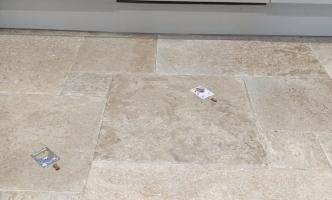Timber using my swaps to wrongly predict the first match

### Similar posts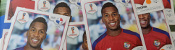World Cup stickers 2018, pt. 2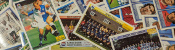World Cup stickers 2018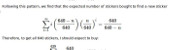World Cup stickersEuro 2016 stickers

### Comments

Comments in green were written by me. Comments in blue were not written by me.
@Matthew: Thank you for the calculations. Good job I ordered the stickers I wanted #IRN. 2453 stickers - that's more than the number you bought (1781) to collect all stickers!
Milad
@Matthew: Here is how I calculated it:

You want a specific set of 20 stickers. Imagine you have already $$n$$ of these. The probability that the next sticker you buy is one that you want is
$$\frac{20-n}{682}.$$
The probability that the second sticker you buy is the next new sticker is
$$\mathbb{P}(\text{next sticker is not wanted})\times\mathbb{P}(\text{sticker after next is wanted})$$
$$=\frac{662+n}{682}\times\frac{20-n}{682}.$$
Following the same method, we can see that the probability that the $$i$$th sticker you buy is the next wanted sticker is
$$\left(\frac{662+n}{682}\right)^{i-1}\times\frac{20-n}{682}.$$
Using this, we can calculate the expected number of stickers you will need to buy until you find the next wanted one:
$$\sum_{i=1}^{\infty}i \left(\frac{20-n}{682}\right) \left(\frac{662+n}{682}\right)^{i-1} = \frac{682}{20-n}$$
Therefore, to get all 682 stickers, you should expect to buy
$$\sum_{n=0}^{19}\frac{682}{20-n} = 2453 \text{ stickers}.$$
Matthew
@Matthew: Yes, I would like to know how you work it out please. I believe I have left my email address in my comment. It seems like a lot of stickers if you are just interested in one team.
Milad
@Milad: Following a similiar method to this blog post, I reckon you'd expect to buy 2453 stickers (491 packs) to get a fixed set of 20 stickers. Drop me an email if you want me to explain how I worked this out.
Matthew
Thanks for the maths, but I have one probability question. How many packs would I have to buy, on average, to obtain a fixed set of 20 stickers?
Milad
Add a Comment

I will only use your email address to reply to your comment (if a reply is needed).

Allowed HTML tags: <br> <a> <small> <b> <i> <s> <sup> <sub> <u> <spoiler> <ul> <ol> <li>
To prove you are not a spam bot, please type "g" then "r" then "a" then "p" then "h" in the box below (case sensitive):
2018-06-16
This year, like every World Cup year, I've been collecting stickers to fill the official Panini World Cup sticker album. Back in March, I calculated that I should expect it to cost £268.99 to fill this year's album (if I order the last 50 stickers). As of 6pm yesterday, I need 47 stickers to complete the album (and have placed an order on the Panini website for these).

### So... How much did it cost?

In total, I have bought 1781 stickers (including the 47 I ordered) at a cost of £275.93. The plot below shows the money spent against the number of stickers stuck in, compared with the what I predicted in March.
To create this plot, I've been keeping track of exactly which stickers were in each pack I bought. Using this data, we can look for a few more things. If you want to play with the data yourself, there's a link at the bottom to download it.

### Swaps

The bar chart below shows the number of copies of each sticker I got (excluding the 47 that I ordered). Unsurprisingly, it looks a lot like random noise.
The sticker I got most copies of was sticker 545, showing Panana player Armando Cooper.
I got swaps of 513 different stickers, meaning I'm only 169 stickers short of filling a second album.

### First pack of all swaps

Everyone who has every done a sticker book will remember the awful feeling you get when you first get a pack of all swaps. For me, the first time this happened was the 50th pack. The plot below shows when the first pack of all swaps occurred in 500,000 simulations.
Looks like I was really quite unlucky to get a pack of all swaps so soon.

### Duplicates in a pack

In all the 345 packs that I bought, there wasn't a single pack that contained two copies of the same sticker. In fact, I don't remember ever getting two of the same sticker in a pack. For a while I've been wondering if this is because Panini ensure that packs don't contain duplicates, or if it's simply very unlikely that they do.
If it was down to unlikeliness, the probability of having no duplicates in one pack would be:
\begin{align} \mathbb{P}(\text{no duplicates in a pack}) &= 1 \times\frac{681}{682}\times\frac{680}{682}\times\frac{679}{682}\times\frac{678}{682}\\ &= 0.985 \end{align}
and the probability of none of my 345 containing a duplicate would be:
\begin{align} \mathbb{P}(\text{no duplicates in 345 packs}) &= 0.985^{345}\\ &= 0.00628 \end{align}
This is very very small, so it's safe to conclude that Panini do indeed ensure that packs do not contain duplicates.

### The data

If you'd like to have a play with the data yourself, you can download it here. Let me know if you do anything with it...

### Similar posts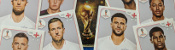World Cup stickers 2018, pt. 3World Cup stickers 2018World Cup stickersEuro 2016 stickers

### Comments

Comments in green were written by me. Comments in blue were not written by me.
Add a Comment

I will only use your email address to reply to your comment (if a reply is needed).

Allowed HTML tags: <br> <a> <small> <b> <i> <s> <sup> <sub> <u> <spoiler> <ul> <ol> <li>
To prove you are not a spam bot, please type "x" then "-" then "a" then "x" then "i" then "s" in the box below (case sensitive):
2018-05-02
Every morning just before 7am, one of the Today Programme's presenters reads out a puzzle. Yesterday, it was this puzzle:In a given month, the probability of a certain daily paper either running a story about inappropriate behaviour at a party conference or running one about somebody's pet being retrieved from a domestic appliance is exactly half the probability of the same paper containing a photo of a Tory MP jogging. The probability of no such photo appearing is the same as that of there being a story about inappropriate behaviour at a party conference. The probability of the paper running a story about somebody's pet being retrieved from a domestic appliance is a quarter that of its containing a photo of a Tory MP jogging. What are the probabilities the paper will (a) run the conference story, (b) run the pet story, (c) contain the jogging photo?I'm not the only one to notice that some of Radio 4's daily puzzles are not great. I think this puzzle is a great example of a terrible puzzle. You can already see the first problem with it: it's long and words and very hard to follow on the radio. But maybe this isn't so important, as you can read it here after it's been read out.
Once you've done this, you can re-write the puzzle as follows: there are three news stories ($$A$$, $$B$$ and $$C$$) that the newspaper might publish in a month. We are given the following information:
$$\mathbb{P}(A\text{ or }B)=\tfrac12\mathbb{P}(C)$$ $$1-\mathbb{P}(C)=\mathbb{P}(A)$$ $$\mathbb{P}(B)=\tfrac14\mathbb{P}(C)$$
To solve this puzzle, we need use the formula $$\mathbb{P}(A\text{ or }B)=\mathbb{P}(A)+\mathbb{P}(B)-\mathbb{P}(A\text{ and }B)$$. These Venn diagrams justify this formula:Venn diagrams showing that $$\mathbb{P}(A\text{ or }B)=\mathbb{P}(A)+\mathbb{P}(B)-\mathbb{P}(A\text{ and }B)$$.
Using the information we were given in the question, we get:
\begin{align} \tfrac12\mathbb{P}(C)&=\mathbb{P}(A\text{ or }B)\\ &=1-\mathbb{P}(C)+\tfrac14\mathbb{P}(C)-\mathbb{P}(A\text{ and }B)\\ \mathbb{P}(C)&=\tfrac45(1-\mathbb{P}(A\text{ and }B)). \end{align}
At this point we have reached the second problem with this puzzle: there's no answer unless we make some extra assumptions, and the question doesn't make it clear what we can assume. But let's give the puzzle the benefit of the doubt and try some assumptions.

### Assumption 1: The events are mutually exclusive

If we assume that the events $$A$$ and $$B$$ are mutually exclusive—or, in other words, only one of these two articles can be published, perhaps due to a lack of space—then we can use the fact that
$$\mathbb{P}(A\text{ and }B)=0.$$
This means that $$\mathbb{P}(C)=\tfrac45$$, $$\mathbb{P}(A)=\tfrac15$$, and $$\mathbb{P}(B)=\tfrac15$$. There's a problem with this answer, though: the three probabilities add up to more than 1.
This wouldn't be a problem, except we assumed that only one of the articles $$A$$ and $$B$$ could be published. The probabilities adding up to more than 1 means that either $$A$$ and $$C$$ are not mutually exclusive or $$A$$ and $$B$$ are not mutually exclusive, so $$C$$ could be published alongside $$A$$ or $$B$$. There seems to be nothing special about the three news stories to mean that only some combinations could be published together, so at this point I figured that this assumption was wrong and moved on.
Today, however, the answer was posted, and this answer was given (without an working out). So we have a third problem with this puzzle: the answer that was given is wrong, or at the very best is based on questionable assumptions.

### Assumption 2: The events are independent

If we assume that the events are independent—so one article being published doesn't affect whether or not another is published—then we may use the fact that
$$\mathbb{P}(A\text{ and }B)=\mathbb{P}(A)\mathbb{P}(B).$$
If we let $$c=\mathbb{P}(C)$$, then we get:
\begin{align} \tfrac12c&=\mathbb{P}(A)+\mathbb{P}(B)-\mathbb{P}(A\text{ and }B)\\ &=\mathbb{P}(A)+\mathbb{P}(B)-\mathbb{P}(A)\mathbb{P}(B)\\ &=1-c+\tfrac14c-\tfrac14(1-c)c\\ \tfrac14c^2-\tfrac32c+1&=0. \end{align}
You can use your favourite formula to solve this to find that $$c=3-\sqrt5$$, and therefore $$\mathbb{P}(A)=\sqrt5-2$$ and $$\mathbb{P}(B)=\tfrac34-\tfrac{\sqrt5}4$$.
In this case, our assumption appears to be more reasonable—as over the course of a month the stories published by a paper probably don't have much of an effect on each other—but we have the fourth, and probably biggest problem with the puzzle: the question and answer are not interesting or surprising, and the method is a bit tedious.

### Similar postsWorld Cup stickers 2018, pt. 3World Cup stickers 2018, pt. 2A 20,000-to-1 baby?World Cup stickers 2018

### Comments

Comments in green were written by me. Comments in blue were not written by me.
@Stefan: It's possible that that was intended but it's not completely clear. If that was the intention, I stick to my point that it's odd that the other story can be published alongside these two.
Matthew
Doesn’t the word ‘either’ in the opening phrase make A and B explicity exclusive?
Stefan
Add a Comment

I will only use your email address to reply to your comment (if a reply is needed).

Allowed HTML tags: <br> <a> <small> <b> <i> <s> <sup> <sub> <u> <spoiler> <ul> <ol> <li>
To prove you are not a spam bot, please type "d" then "e" then "c" then "a" then "g" then "o" then "n" in the box below (case sensitive):
2018-03-23
This morning, I heard about Arnie Ellis on the Today programme. Arnie is the first baby boy to be born in his family in five generations, following ten girls. According to John Humphrys, there is a 20,000-to-1 chance of this happening. Pretty quickly, I started wondering where this number came from.
After a quick Google, I found that this news story had appeared in many of today's papers, including the Sun and the Daily Mail. They all featured this 20,000-to-1 figure, which according to The Sun originally came from Ladbrokes.

### What is the chance of this happening?

If someone is having a child, the probability of it being a girl is 0.5. The probability of it being a boy is also 0.5. So the probaility of having ten girls followed by a boy is
$$\left(\tfrac12\right)^{10}\times\tfrac12=\frac1{2048}.$$
If all 11 children were siblings, then this would be the chance of this happening—and it's a long way off the 20,000-to-1. But in Arnie's case, the situation is different. Luckily in the Daily Mail article, there is an outline of Arnie's family tree.
Here, you can see that the ten girls are spread over five generations. So the question becomes: given a baby, what is the probability that the child is male and his most recently born ten relatives on their mother's side are all female?
Four of the ten relatives are certainly female—Arnie's mother, grandmother, great grandmother and great great grandmother are all definitely female. This only leaves six more relatives, so the probability of a baby being in Arnie's position is
$$\left(\tfrac12\right)^{6}\times\tfrac12=\frac1{128}.$$
This is now an awful lot lower than the 20,000-to-1 we were told. In fact, with around 700,000 births in the UK each year, we'd expect over 5,000 babies to be born in this situation every year. Maybe Arnie's not so rare after all.
This number is based on the assumption that the baby's last ten relatives are spread across five generations. But the probability will be different if the relatives are spread over a different number of generations. Calculating the probability for a baby with any arrangement of ancestors would require knowing the likelihood of each arrangement of relatives, which would require a lot of data that probably doesn't exist. But the actual anwer is probably not too far from 127-to-1.

### Where did 20,000-to-1 come from?

This morning, I emailed Ladbrokes to see if they could shed any light on the 20,000-to-1 figure. They haven't got back to me yet. (Although they did accidentally CC me when sending the query on to someone who might know the answer, so I'm hopeful.) I'll update this post with an explanaation if I do hear back.
Until then, there is one possible explanation for the figure: we have looked at the probability that a baby will be in this situation, but we could instead have started at the top of the family tree and looked at the probability that Beryl's next ten decendents were girls followed by a boy. The probability of this happening will be lower, as there is a reasonable chance that Beryl could have no female children, or no children at all. Looking at the problem this way, there are more ways for the situation to not happen, so the probability of it happening is lower.
But working the actually probability out in this way would again require data about how many children are likely in each generation, and would be a complicated calculation. It seems unlikely that this is what Ladbrokes did. Let's hope they shed some light on it...

### Similar postsWorld Cup stickers 2018, pt. 3World Cup stickers 2018, pt. 2A bad Puzzle for TodayWorld Cup stickers 2018

### Comments

Comments in green were written by me. Comments in blue were not written by me.
Add a Comment

I will only use your email address to reply to your comment (if a reply is needed).

Allowed HTML tags: <br> <a> <small> <b> <i> <s> <sup> <sub> <u> <spoiler> <ul> <ol> <li>
To prove you are not a spam bot, please type "equation" in the box below (case sensitive):
2018-03-22
Back in 2014, I worked out the cost of filling an official Panini World Cup 2014 sticker book. Today, the 2018 sticker book was relased: compared to four years ago, there are more stickers to collect and the prices have all changed. So how much should we expect it to cost us to fill the album this time round?

### How many stickers will I need?

There are 682 stickers to collect. Imagine you have already stuck $$n$$ stickers into your album. The probability that the next sticker you buy is new is
$$\frac{682-n}{682}.$$
The probability that the second sticker you buy is the next new sticker is
$$\mathbb{P}(\text{next sticker is not new})\times\mathbb{P}(\text{sticker after next is new})$$ $$=\frac{n}{682}\times\frac{682-n}{682}.$$
Following the same method, we can see that the probability that the $$i$$th sticker you buy is the next new sticker is
$$\left(\frac{n}{682}\right)^{i-1}\times\frac{682-n}{682}.$$
Using this, we can calculate the expected number of stickers you will need to buy until you find a new one:
$$\sum_{i=1}^{\infty}i \left(\frac{682-n}{682}\right) \left(\frac{n}{682}\right)^{i-1} = \frac{682}{682-n}$$
Therefore, to get all 682 stickers, you should expect to buy
$$\sum_{n=0}^{681}\frac{682}{682-n} = 4844 \text{ stickers}.$$

### How much will this cost?

You can buy the following [source]:
• Starter pack (an album and 31 stickers) for £3.99
• Sticker packs (5 stickers) for 80p
• Sticker multipacks (30 stickers) for £4.50
First of all you'll need to buy the starter pack, as you need an album to stick everything in. This comes with 31 stickers; we should expect to buy 4813 more stickers after this.
The cheapest way to buy these stickers is to buy them in multipacks for 15p per sticker. This gives a total expected cost of filling the sticker album of £725.94. (Although if your local newsagent doesn't stock the multipacks, buying 80p packs to get these stickers will cost you £774.07.)

### What if I order the last 50 stickers?

If you'd like to spend a bit less on the sticker book, Panini lets you order the last 50 stickers to complete your album. This is very helpful as these last 50 stickers are the most expensive.
You can order missing stickers from the Panini website for 22p per sticker's sticker ordering service for the 2018 World Cup doesn't appear to be online yet; but based on other recent collections, it looks like ordered stickers will cost 16p each, with £1 postage per order.
Ordering the last 50 stickers reduces the expected number of other stickers you need to buy to
$$\sum_{n=0}^{631}\frac{682}{682-n} = 1775 \text{ stickers}.$$
This reduces the expected overall cost to £291.03. So I've just saved you £434.91.

### What if I order more stickers?

Of course, if you're willing to completely give up on your morals, you could order more than one batch of 50 stickers from Panini. This raises the question: how many should you order to minimise the expected cost.
If you order the last $$a$$ stickers, then you should expect to pay:
• £3.99 for the album and first 31 stickers
• £$$\displaystyle0.15\left(\sum_{n=1}^{681-a}\frac{682}{682-n}-31\right)$$ for other stickers you need
• £$$\displaystyle \left(0.16a+\left\lceil \frac{a}{50}\right\rceil\right)$$ to order the last $$a$$ stickers
The total expected cost of filling your album for different values of $$a$$ is shown in the graph below.
The red cross shows the point at which the album is cheapest: this is when the last 550 stickers are ordered, giving a total expected cost of £120.18. That's another £170.85 I've saved you. You're welcome.
Still, ordering nearly all stickers to minimise the cost doesn't sound like the most fun way to complete the sticker book, so you probably need to order a few less than this to maximise your fun.

### What about swaps?

Of course, you can also get the cost of filling the book down by swapping your spare stickers with friends. In 2016, I had a go at simulating filling a sticker book with swapping and came to the possibly obvious conclusion that the more friends you have to swap with, the cheaper filling the book will become.
My best advice for you, therefore, is to get out there right now and start convinding your friends to join you in collecting stickers.

### Similar postsWorld Cup stickers 2018, pt. 3World Cup stickers 2018, pt. 2World Cup stickersEuro 2016 stickers

### Comments

Comments in green were written by me. Comments in blue were not written by me.
@RM: It would slightly reduce the expected cost I think, but I need to do some calculations to make sure.

I'm not certain whether they guarantee that there are no duplicates in a pack or just it's very unlikely... Follow up post looking into this coming soon
Matthew
Helpful article!! My head always go into a spine with probability, but does the fact that you would not get a double within a pack affect the overall strategy or cost? My head says this is an another advantage of buying 30 packs over the 5.
RM
Add a Comment

I will only use your email address to reply to your comment (if a reply is needed).

Allowed HTML tags: <br> <a> <small> <b> <i> <s> <sup> <sub> <u> <spoiler> <ul> <ol> <li>
To prove you are not a spam bot, please type "rebmun" backwards in the box below (case sensitive):

## Archive

Show me a random blog post
▼ show ▼
© Matthew Scroggs 2012–2021